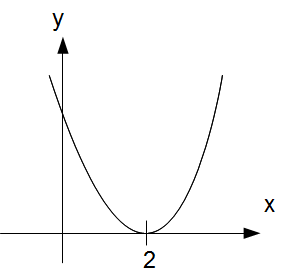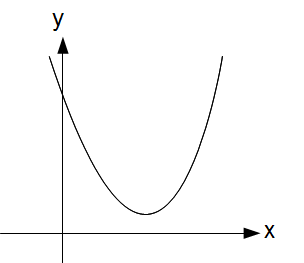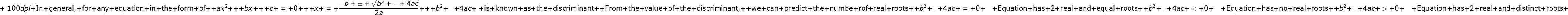### Roots of a Quadratic Equation

What do we mean by the roots of a quadratic equation?

Example 1We say that 2 and 1 are roots of the equation. The equation has 2 real and distinct rootsIn graphical form, the roots of the equation are represented as the intersection of the curve with the x axis.

Example 2In this case there is only 1 solution. We say that the equation has only 2 real and equal roots
Graphically it can be represented asNotice that there is only 1 intersection point between the curve and the x axis

Example 3
Lastly we are going to look at an example where the curve does not intersect the x axis
Consider the equation:The equation cannot be factorized. It cannot be solved using the quadratic formula.
Graphically it is represented asIt turns out that we can predict the number of roots that an equation has by looking at the discriminant1.Remarkable post. I simply came across your blog and desired to say that I have really enjoyed searching your blog posts. Thank you for sharing such blogs. math tuition

2.I am glad to discover this post helpful for me, as it contains a great deal of data. I generally want quality content and this thing, I found in your post. Thank you. academic writing services New York

3.I read your post and got it quite informative. I couldn't find any knowledge on this matter prior to. I would like to thanks for sharing this article here . metro atlanta church